SNVSA56B May   2015  – February 2017

PRODUCTION DATA.

1. Features
2. Applications
3. Description
1.     Device Images
4. Revision History
5. Pin Configuration and Functions
6. Specifications
7. Detailed Description
1. 7.1 Overview
2. 7.2 Functional Block Diagram
3. 7.3 Feature Description
4. 7.4 Device Functional Modes
8. Application and Implementation
1. 8.1 Application Information
2. 8.2 Typical Application - Voltage Inverter
1. 8.2.1 Design Requirements
2. 8.2.2 Detailed Design Requirements
3. 8.2.3 Application Curve
9. Power Supply Recommendations
10. 10Layout
11. 11Device and Documentation Support
12. 12Mechanical, Packaging, and Orderable Information

• DBV|6

### 6.7 Typical Characteristics

(Typical Application circuit, VIN = 3.6 V unless otherwise specified.)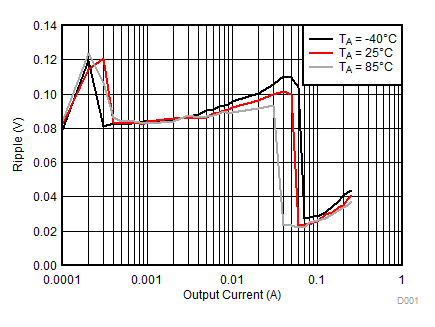VIN = 5.5 V
Figure 1. Output Ripple vs Output Current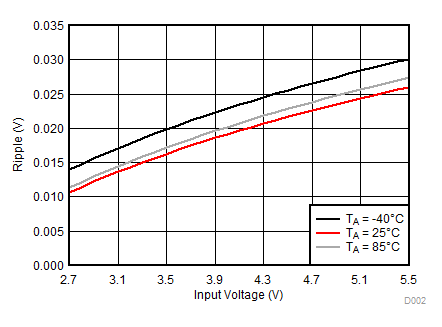IOUT = 100 mA
Figure 2. Output Ripple vs Input Voltage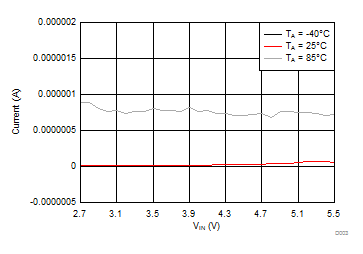Figure 3. Shutdown Current vs Input VoltageVIN = 5.5 V
Figure 5. Output Impedance vs Output Current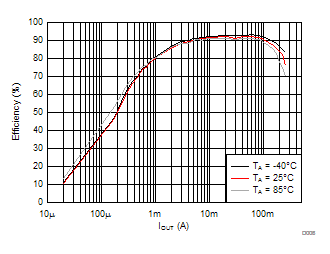VIN = 3.6 V
Figure 7. Efficiency vs Output Current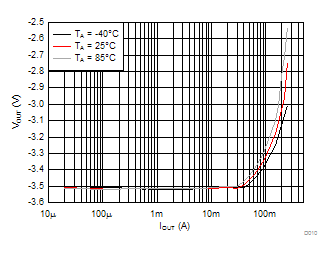VIN = 3.6 V
Figure 9. Output Voltage vs Output Current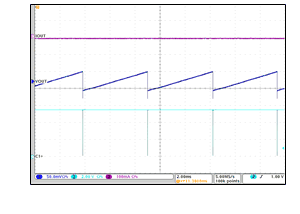IOUT = 0 mA VIN = 5.5 V
Figure 11. Unloaded Output Voltage Ripple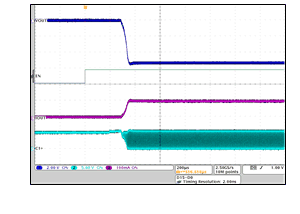EN = 1 VIN = 5.5 V IOUT = 100 mA
Figure 13. EN High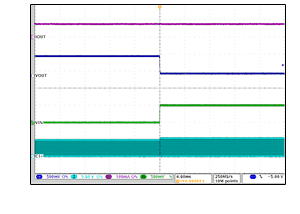IOUT = 75 mA
Figure 15. Line Step 5.5 V to 5 V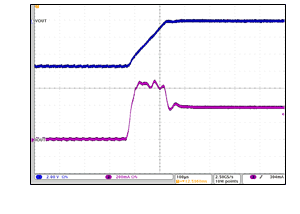VIN = 5.5 V
Figure 17. Output Short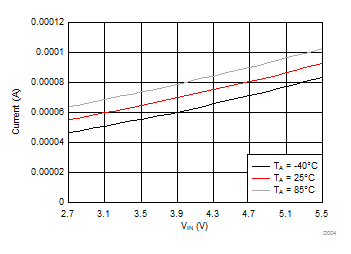Figure 4. Quiescent Current vs Input Voltage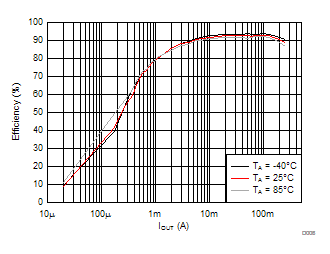VIN = 5.5 V
Figure 6. Efficiency vs Output Current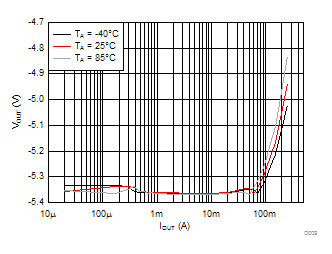VIN = 5.5 V
Figure 8. Output Voltage vs Output CurrentIOUT = 150 mA
Figure 10. Frequency vs Input Voltage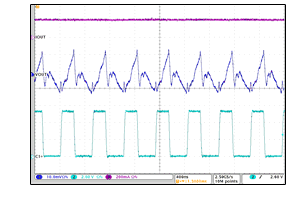IOUT = 200 mA VIN = 5.5 V
Figure 12. Loaded Output Voltage Ripple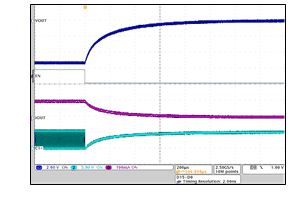EN = 0 VIN = 5.5 V IOUT = 100 mA
Figure 14. EN Low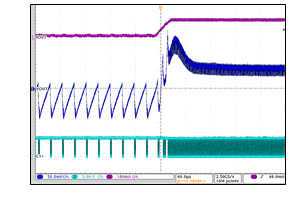VIN = 5.5 V
Figure 16. Load Step 10 mA to 100 mA